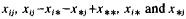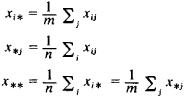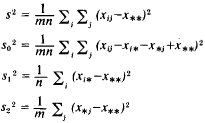# analysis of variance

(redirected from Analysis of variance/Random effects models)
Also found in: Dictionary, Thesaurus, Medical, Financial.

## analysis of variance

[ə¦nal·ə·səs əv ′ver·ē·əns]
(statistics)
A method for partitioning the total variance in experimental data into components assignable to specific sources.
McGraw-Hill Dictionary of Scientific & Technical Terms, 6E, Copyright © 2003 by The McGraw-Hill Companies, Inc.

## analysis of variance (ANOVA)

(STATISTICS) a procedure used to test whether differences between the MEANS of several groups are likely to be found in the population from which those groups were drawn. An example might be three groups of people with different educational backgrounds for whom the mean wage level has been calculated. ANOVA provides a way of testing whether the differences between the means are statistically significant by dividing the variability of the observations into two types. One type, called ‘within group’ variability, is the VARIANCE within each group in the SAMPLE. The second type is the variability between the group means (‘between groups’ variability). If this is large compared to the ‘within group’ variability it is likely that the population’s means are not equal.

The assumptions underlying the use of analysis of variance are:

1. each group must be a RANDOM SAMPLE from a normal population (see NORMAL DISTRIBUTION);
2. the variance of the groups in the population are equal.

However, the technique is robust and can be used even if the normality and equal variance assumptions do not hold. The random sample condition is nevertheless essential. See also SIGNIFICANCE TEST.

Collins Dictionary of Sociology, 3rd ed. © HarperCollins Publishers 2000
The following article is from The Great Soviet Encyclopedia (1979). It might be outdated or ideologically biased.

## Analysis of Variance

a statistical method in mathematics for determining the effect of separate factors on the result of an experiment. Analysis of variance was first proposed by the British statistician R. A. Fisher in 1925 for analyzing results of agricultural experiments designed to reveal under what conditions a specific agricultural crop provided a maximum yield. Modern applications of analysis of variance encompass a broad range of problems in economics, biology, and technology and are generally interpreted in terms of a statistical theory for determining systematic variations among the results of measurements made under the influence of several varying factors.

If the values of the unknown constants a1, … , an could be measured by various methods or means of measurement M1, …, Mm and, in each instance, a systematic error could depend on the selected method as well as on the unknown value of ai being measured, then the results of the measurements xij are represented as sums of the form

xij = ai + bij + δiji 1, 2, ... , = n; j = 1, 2, ... , m

where bij is a systematic error arising during the measurement of a1 by method Mi, and δij is a random error. Such a model is called a two-factor layout of analysis of variance (the first factor is the quantity being measured and the second, the method of measurement). Variances in the empirical distributions, corresponding to the sets of random valueswhereare expressed by the formulasThese variances satisfy the identity =

s2 = s02 + s12 + s22

which also explains the origin of the term “analysis of variance.”

If the values of systematic errors do not depend on the method of measurement (that is, there are no systematic variations among the methods of measurement), then the ratio s22/s02 is close to 1. This property is the basic criterion for the statistical determination of systematic variations. If s22 differs significantly from 1, then the hypothesis about the absence of systematic variations is rejected. The significance of the difference is determined according to the probability distribution of the random errors in the measurements. Specifically, if all measurements are of equal accuracy and the random errors are normally distributed, then the critical values for the ratio s22/s02 are determined by F -distribution tables (distribution of the variance ratio).

The above scheme allows only for detecting the existence of systematic variations and, generally speaking, is not suitable for their numerical evaluation with subsequent elimination from the observed results. Such evaluation may be achieved only through numerous measurements (with repeated applications of the described scheme).

### REFERENCES

Scheffe, H. Dispersionnyi analiz. Moscow, 1963. (Translated from English.)
Smirnov, N. V., and I. V. Dunin-Barkovskii. Kurs teorii veroiatnostei i matematicheskoi statistiki dlia tekhnicheskikh prilozhenii, 2nd ed. Moscow, 1965.

L. N. BOL’SHEV